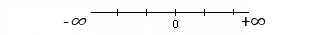# Infinity: Countable, UncountableIs infinity a number? The simple answer is no. Infinity is a concept, an idea that is related to numbers and counting systems; but it is not a number.

The infinity symbol (∞) is a lazy eight curve— an eight lying on its side (not to be confused with “the” eight curve). An easy way to associate this mentally with the concept of infinity is to realize that you can travel endlessly around the curve— there is no beginning and no end. That’s what the concept of infinity means— something without bound, larger than any natural numbers.

## If Infinity it isn’t a number, what is it?

A common definition for infinity is that it’s a quantity bigger than any number. That isn’t quite correct, but it’s good enough for most purposes.

Which exact definition you use depends on which specific area of math you’re working with. For example, if you’re looking at a number line, positive infinity is the last possible “number” to the left; negative infinity is always the last possible “number” to the far right.In set theory, things get a little more complicated, because sets can have countable infinity, or uncountable infinity. Countable infinity is when it’s easy to label the numbers (like if you’re counting whole numbers 1, 2, 3…. Uncountable infinity is where the set contains all the numbers and everything in between, like 1, 1.22, 1.23, 1.256…. Set theory is beyond the scope of this article but if you’re interested in reading more on countable and uncountable sets, read Countable and Uncountable Sets.

## Is Infinity a Number if It’s Transfinite?

Although infinity is not a number, there are a special class of numbers sometimes referred to as “infinite numbers” which are bigger than all finite numbers. More properly, these are called transfinite numbers. So, if a number falls into the “transfinite infinity” class, then yes— it’s a number.

Although infinite numbers— i.e, the transfinites— are sometimes called numbers, it’s important to realize this does not mean they are natural numbers, real numbers, or any other type of number that we are used to working with and manipulating. The Peano axioms, for instance, the basic rules which guide the way we add, subtract, and understand real numbers, do not apply in any way to the transfinites.

The infinite (transfinite) numbers each have their own designation. The first transfinite cardinal number is written as Ν0, which we read as Aleph-naught. This represents the cardinality of the infinite set of natural numbers— the number of elements in that set. The next cardinal number is Ν1.

## The Fear of Infinity

In his book, Mathematics and Its History, J. Stillwell describes how most mathematicians didn’t warm to the concept of infinity until well after the 19th century.

“The infinitude of a process, collection, or magnitude was understood as
the possibility of its indefinite continuation, and no more—certainly not
the possibility of eventual completion.”

For example, the numbers 1, 2, 3, … imply that you can keep on counting forever, without reaching an end. And the sequence x1, x2, … x3 implies the same. Yet even the ancient Greeks were afraid to draw any conclusion about processes having a limit; They were frightened off the subject of limits by Zeno’s paradoxes. However, infinite processes made an appearance during the time of the Pythagoreans. As an example, the recurrence relations for generating integer solutions for x2 – 2y2 = ±1:

• xn+1 = xn + 2yn,
• yn+1 = xn + yn.

However, to avoid the problem surrounding a limiting process, the Greeks simply eliminate those possible solutions—stating instead that some number was “too big” or “too small” to be a solution. The 17th-century saw some “dubious” methods of infinitesimals, and it wasn’t until 19th century mathematicians like Richard Dedekind and Karl Weierstrass, restored the rigorous mathematical standards of the Greeks and infinity became widely accepted as a concept.

## References for Is Infinity a Number?

Rucker, Rudy. The Science and Philosophy of the Infinite. Bantum Books. Retrieved from